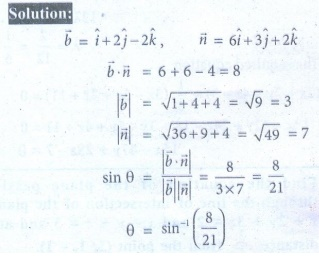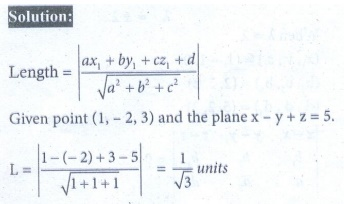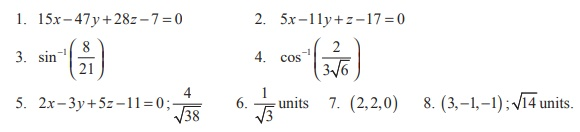Home | | Maths 12th Std | Exercise 6.9: Equation of intersection of the planes

# Exercise 6.9: Equation of intersection of the planes

Maths Book back answers and solution for Exercise questions - Find the equation of the plane passing through the line of intersection of the planes

EXERCISE 6.9

1. Find the equation of the plane passing through the line of intersection of the planes(2ˆ- 7ˆ+ 4ˆ) = 3 and 3- 5 4+11 = 0 , and the point (-2,1, 3) .2. Find the equation of the plane passing through the line of intersection of the planes x + 2 y + 3z = and +11 = 3 , and at a distance 2/√3 from the point (3,1, -1).3. Find the angle between the line= (2ˆi - ˆj + ˆk )+t(ˆi + 2ˆj - ˆkand the plane. (6ˆi + 3ˆj + 2ˆk ) = 84. Find the angle between the planes= (ˆi + ˆj - k ) = 3 and 2x - 2 y + z = 2 .5. Find the equation of the plane which passes through the point (3, 4, -1) and is parallel to the plane 2x - 3y + 5z + 7 = 0 . Also, find the distance between the two planes.6. Find the length of the perpendicular from the point (1, -2, 3) to the plane = 5 .7. Find the point of intersection of the line x -1 = y/2 = z +1 with the plane 2x - y + 2z = 2 . Also, find the angle between the line and the plane.8. Find the coordinates of the foot of the perpendicular and length of the perpendicular from the point ( 4,3,2) to the plane x + 2 y + 3z = 2 .